# Langmuir Sheaths

Virtually all terrestrial plasmas are contained within solid vacuum vessels. But, what happens to plasma in the immediate vicinity of a vessel wall? Actually, to a first approximation, when ions and electrons hit a solid surface they recombine and are lost to the plasma. Hence, we can treat the wall as a perfect sink of particles. Now, given that the electrons in a plasma generally move much faster than the ions, the initial electron flux into the wall greatly exceeds the ion flux, assuming that the wall starts off unbiased with respect to the plasma. Of course, this flux imbalance causes the wall to charge up negatively, and so generates a potential barrier that repels the electrons, and thereby reduces the electron flux. Debye shielding confines this barrier to a thin layer of plasma, whose thickness is a few Debye lengths, coating the inside surface of the wall. This layer is known as a plasma sheath or a Langmuir sheath. The height of the potential barrier continues to grow as long as there is a net flux of negative charge into the wall. This process presumably comes to an end, and a steady-state is attained, when the potential barrier becomes sufficiently large to make electron flux equal to the ion flux (Hazeltine and Waelbroeck 2004).

Let us construct a one-dimensional model of an unmagnetized, steady-state, Langmuir sheath. Suppose that the wall lies at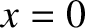, and that the plasma occupies the region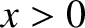. Let us treat the ions and the electrons inside the sheath as collisionless fluids. The ion and electron equations of motion are thus written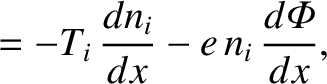(4.243)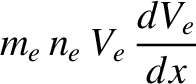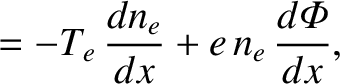(4.244)

respectively. Here,is the electrostatic potential. Moreover, we have assumed uniform ion and electron temperatures,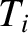and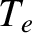, respectively, for the sake of simplicity. We have also neglected any off-diagonal terms in the ion and electron stress-tensors, because these terms are comparatively small. Note that quasi-neutrality does not apply inside the sheath, and so the ion and electron number densities,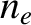and, respectively, are not necessarily equal to one another.

Consider the ion fluid. Let us assume that the mean ion velocity,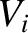, is much greater than the ion thermal velocity,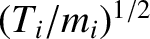. Because, as will become apparent,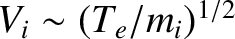, this ordering necessarily implies that: that is, that the ions are cold with respect to the electrons. It turns out that plasmas in the immediate vicinity of solid walls often have comparatively cold ions, so our ordering assumption is fairly reasonable. In the cold ion limit, the pressure term in Equation (4.243) is negligible, and the equation can be integrated to give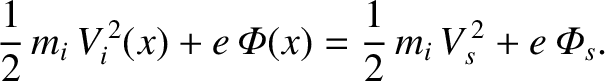(4.245)

Here,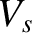and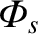are the mean ion velocity and electrostatic potential, respectively, at the edge of the sheath (i.e.,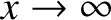). Now, ion fluid continuity requires that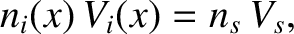(4.246)

whereis the ion number density at the sheath boundary. Incidentally, because we expect quasi-neutrality to hold in the plasma outside the sheath, the electron number density at the edge of the sheath must also be(assuming singly-charged ions). The previous two equations can be combined to give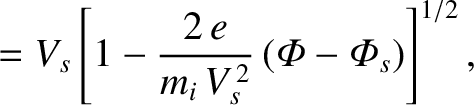(4.247)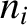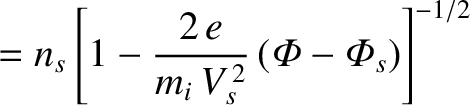(4.248)

Consider the electron fluid. Let us assume that the mean electron velocity,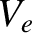, is much less than the electron thermal velocity,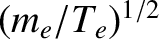. In fact, this must be the case, otherwise, the electron flux to the wall would greatly exceed the ion flux. Now, if the electron fluid is essentially stationary then the left-hand side of Equation (4.244) is negligible, and the equation can be integrated to give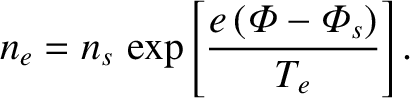(4.249)

Here, we have made use of the fact that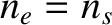at the edge of the sheath.

Poisson's equation is written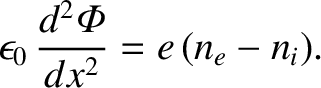(4.250)

It follows that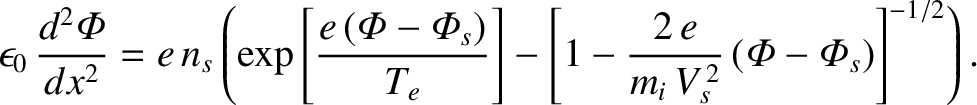(4.251)

Let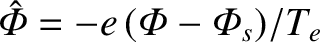,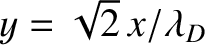, and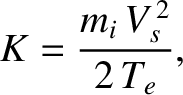(4.252)

where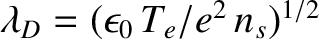is the Debye length. Equation (4.251) transforms to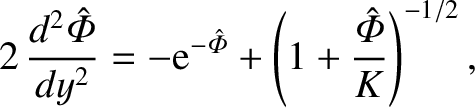(4.253)

subject to the boundary condition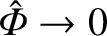as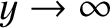. Multiplying through by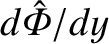, integrating with respect to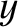, and making use of the boundary condition, we obtain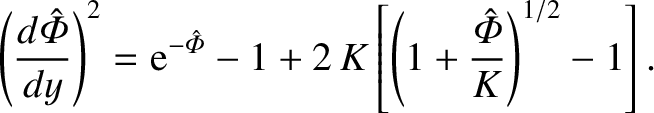(4.254)

Unfortunately, the previous equation is highly nonlinear, and can only be solved numerically. However, it is not necessary to attempt this to see that a physical solution can only exist if the right-hand side of the equation is positive for all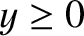. Consider the limit. It follows from the boundary condition that. Expanding the right-hand side of Equation (4.254) in powers of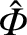, we find that the zeroth- and first-order terms cancel, and we are left with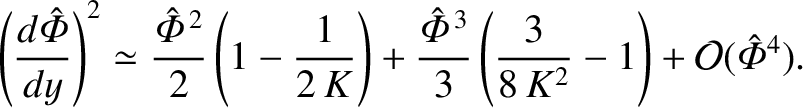(4.255)

Now, the purpose of the sheath is to shield the plasma from the wall potential. It can be seen, from the previous expression, that the physical solution with maximum possible shielding corresponds to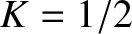, because this choice eliminates the first term on the right-hand side (thereby makingas small as possible at large) leaving the much smaller, but positive (note thatis positive), second term. Hence, we conclude that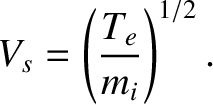(4.256)

This result is known as the Bohm sheath criterion. It is a somewhat surprising result, because it indicates that ions at the edge of the sheath are already moving toward the wall at a considerable velocity. Of course, the ions are further accelerated as they pass through the sheath. Because the ions are presumably at rest in the interior of the plasma, it is clear that there must exist a region sandwiched between the sheath and the main plasma in which the ions are accelerated from rest to the Bohm velocity,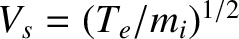. This region is called the pre-sheath, and is both quasi-neutral and much wider than the sheath (the actual width depends on the nature of the ion source).

The ion current density at the wall is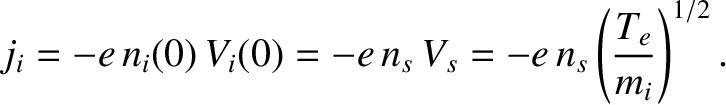(4.257)

This current density is negative because the ions are moving in the negative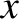-direction. What about the electron current density? Well, the number density of electrons at the wall is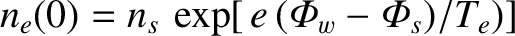, where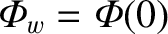is the wall potential. Let us assume that the electrons have a Maxwellian velocity distribution peaked at zero velocity (because the electron fluid velocity is much less than the electron thermal velocity). It follows that half of the electrons atare moving in the negative-direction, and half in the positive-direction. Of course, the former electrons hit the wall, and thereby constitute an electron current to the wall. This current is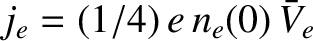, where the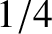comes from averaging over solid angle, and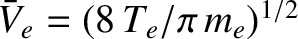is the mean electron speed corresponding to a Maxwellian velocity distribution (Reif 1965). Thus, the electron current density at the wall is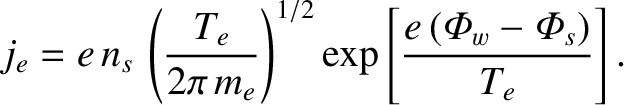(4.258)

In order to replace the electrons lost to the wall, the electrons must have a mean velocity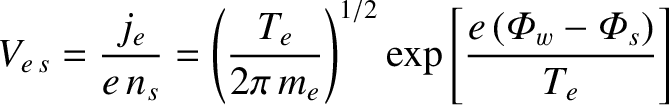(4.259)

at the edge of the sheath. However, we previously assumed that any electron fluid velocity was much less than the electron thermal velocity,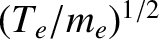. As is clear from the previous equation, this is only possible provided that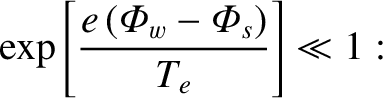(4.260)

that is, provided that the wall potential is sufficiently negative to strongly reduce the electron number density at the wall. The net current density at the wall is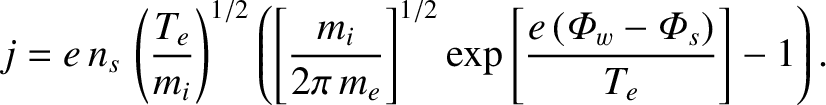(4.261)

Of course, we require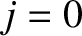in a steady-state sheath, in order to prevent wall charging, and so we obtain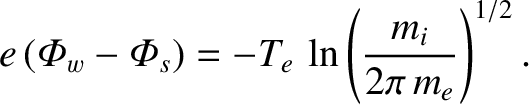(4.262)

We conclude that, in a steady-state sheath, the wall is biased negatively with respect to the sheath edge by an amount that is proportional to the electron temperature.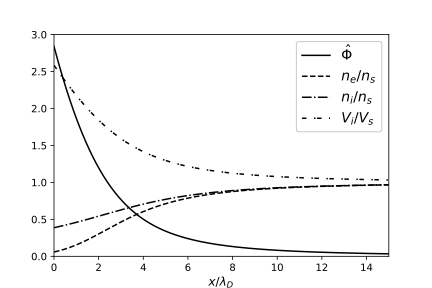For a hydrogen plasma,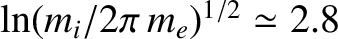. Thus, hydrogen ions enter the sheath with an initial energy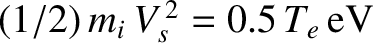, fall through the sheath potential, and so impact the wall with energy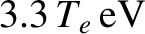.

Combining Equations (4.247)–(4.249), (4.254), (4.262), and making use of the constraint, we arrive at the following set of equations that characterize the structure of a Langmuir sheath: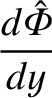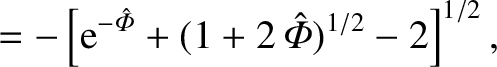(4.263)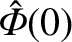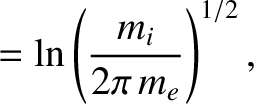(4.264)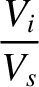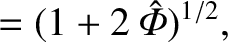(4.265)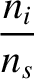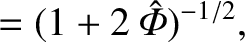(4.266)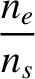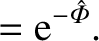(4.267)

Equation (4.263) can be solved numerically, subject to the boundary condition (4.264), to give the results summarized in Figure 4.4. The figure illustrates how the deviation from quasi-neutrality within the sheath generates an electric potential that greatly reduces the electron number density at the wall, and also accelerates the ions as they pass through the sheath (toward the wall).